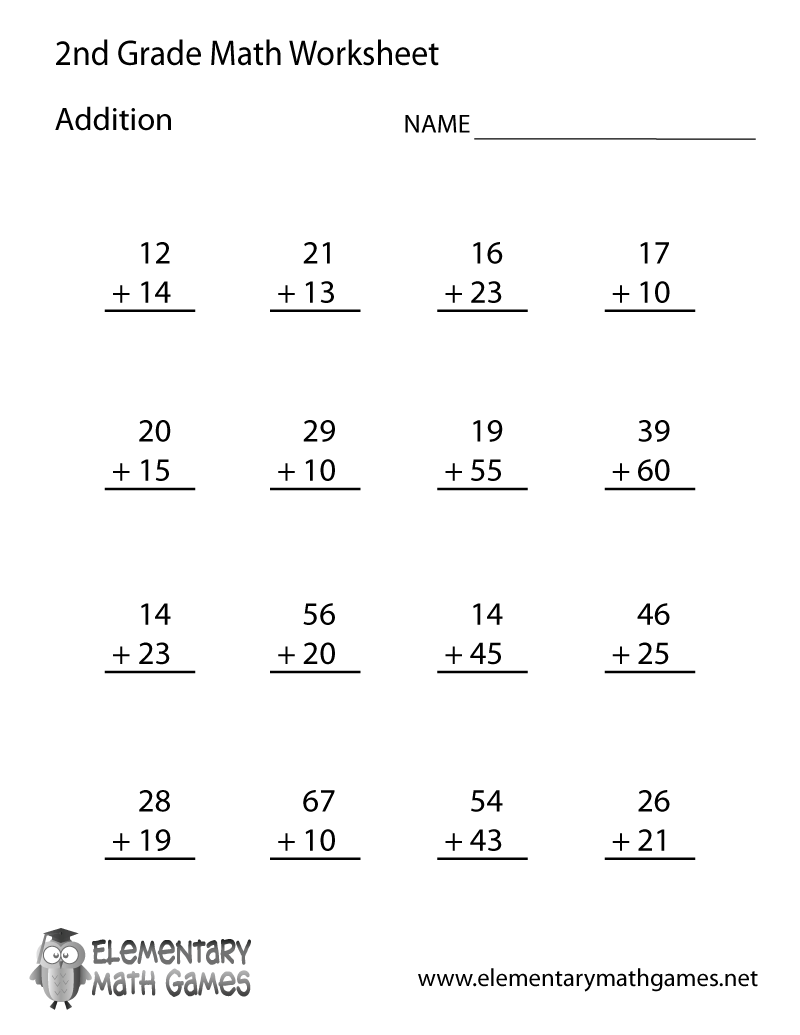## Learn and Practice Addition

This second grade math worksheet is a great way for students to learn how to add two digit numbers. There are 16 addition problems for second graders to solve.

Here are the two versions of this free second grade math worksheet: